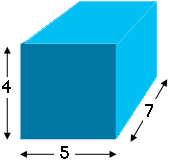SEARCH HOMEMath Central Quandaries & QueriesQuestion from Manraj, a student: Find the surface area of a rectangular prism with dimensions 4, 5, and 7 units.Hi Manraj,

Draw a diagram and label the lengths of the edges. (My diagram is not to scale.)How many faces are there? What are the dimensions of each face? What is the area of each face? What is the total area of the faces?

PennyMath Central is supported by the University of Regina and The Pacific Institute for the Mathematical Sciences.